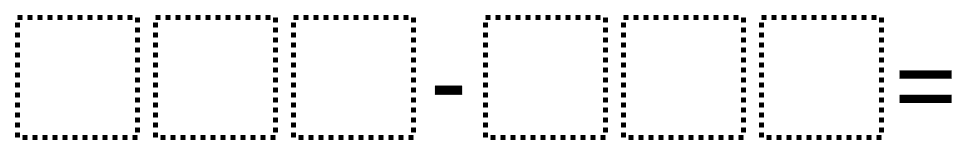# Subtracting 3-Digit Numbers 2

Directions: Using the digits 1 to 9 at most one time each, place a digit in the boxes to make a difference that is as close to 329 as possible.### Hint

What strategies might you use to find the numbers? (Possibilities including subtracting as well as adding up).

There are many possible answers including 871 – 542.

Source: Robert Kaplinsky

## Multiply and Divide Within A Hundred 1

Directions: Using the digits 2 to 9 at most one time each, place a digit …

1.2.3.4.5.6.